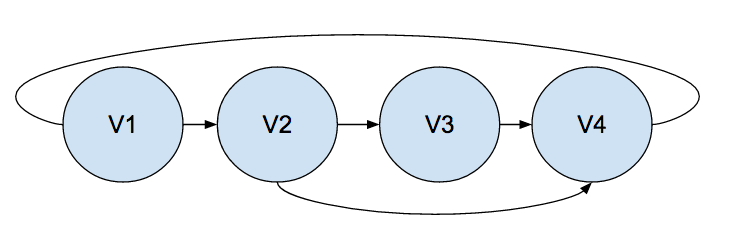Erlang Thursday – digraph:del_path/3

Today’s Erlang Thursday is on digraph:del_path/3.

We will continue working with the same graph we started with in the previous post on digraph:get_path/3.Graph = digraph:new().
% {digraph,20498,24595,28692,true}
% ['\$v'|0]
% ['\$v'|1]
% ['\$v'|2]
% ['\$v'|3]
% ['\$e'|0]
% ['\$e'|1]
% ['\$e'|2]
% ['\$e'|3]
% ['\$e'|4]

digraph:del_path/3 takes three arguments, a Graph, a source vertex, and a destination vertex, and removes all edges in a each path in the graph from the source vertex to the destination vertex, until no path exist between the source and destination vertices.

The return value of digraph:del_path/3 is always a return value of true.

Looking at the picture of the graph above as reference, we are going to call digraph:del_path/3 for the graph with a source vertex of V1, and a destination vertex of V4.

digraph:del_path(Graph, V1, V4).
% true
digraph:vertices(Graph).
% [['\$v'|2],['\$v'|1],['\$v'|0],['\$v'|3]]
digraph:edges(Graph).
% [['\$e'|1],['\$e'|2],['\$e'|4]]

Translating the edge names, we see that the edge from V1 to V2 has been removed, as well as the edge from V2 to V4 has been removed.

So how did Erlang come up with this result?

This puzzled me at first, as it wasn’t one of the two scenarios I was expecting to see, which were either: remove all edges but the edge from V4 to V1, or remove only the edge from V1 to V2.

I then opened the Erlang source code on Github for digraph module to clarify my thinking, and looking at the code it then made sense what was happening.

First digraph:del_path/3 calls digraph:get_path/3, and removes all edges in that path, and then recurses until no path is found.

This is when it clicked as to why Erlang was removing only those edges.

If we call digraph:get_path/3 on a fresh version of the graph, we see that it returns the path of V1 -> V2 -> V4.

digraph:get_path(Graph, V1, V4).
[['\$v'|0],['\$v'|1],['\$v'|3]]

Erlang then removes the edges in that path, and the will call digraph:del_path/3, which calls digraph:get_path/3 again, but as we removed the edge between V1 and V2, no path is found so the process is finished.

This is why we see more edges removed if we reset the Graph again (by exiting the shell and recreating it from scratch by pasting the initialization into the shell), and call digraph:del_path/3 between V2 and V4.

digraph:del_path(Graph, V2, V4).
% true
digraph:edges(Graph).
% [['\$e'|0],['\$e'|4]]

This case there are the paths V2 -> V4 and V2 -> V3 -> V4, and if we remove the path V2 -> V4, the removal of all the edges associated with that path doesn’t break the path of V2 -> V3 -> V4, so it can remove all edges in that path as well.

So we have a win in the case where the documentation wasn’t quite as clear as it could be, but having the Erlang standard library be open source gets us a win because we can always go in and check out what the code is really doing.

–Proctor

This site uses Akismet to reduce spam. Learn how your comment data is processed.